## Heegner Number

The values offor which Quadratic Fieldsare uniquely factorable into factors of the form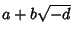. Here,andare half-integers, except for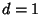and 2, in which case they are Integers. The Heegner numbers therefore correspond to Discriminantswhich have Class Number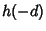equal to 1, except for Heegner numbersand, which correspond toand, respectively.

The determination of these numbers is called Gauss's Class Number Problem, and it is now known that there are only nine Heegner numbers:,,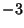,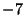,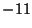,,,, and(Sloane's A003173), corresponding to discriminants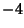,,,,,,,, and, respectively.

Heilbronn and Linfoot (1934) showed that if a largerexisted, it must be. Heegner (1952) published a proof that only nine such numbers exist, but his proof was not accepted as complete at the time. Subsequent examination of Heegner's proof show it to be essentially'' correct (Conway and Guy 1996).

The Heegner numbers have a number of fascinating connections with amazing results in Prime Number theory. In particular, the j-Function provides stunning connections between,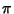, and the Algebraic Integers. They also explain why Euler's Prime-Generating Polynomial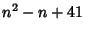is so surprisingly good at producing Primes.

References

Conway, J. H. and Guy, R. K. The Nine Magic Discriminants.'' In The Book of Numbers. New York: Springer-Verlag, pp. 224-226, 1996.

Heegner, K. Diophantische Analysis und Modulfunktionen.'' Math. Z. 56, 227-253, 1952.

Heilbronn, H. A. and Linfoot, E. H. On the Imaginary Quadratic Corpora of Class-Number One.'' Quart. J. Math. (Oxford) 5, 293-301, 1934.

Sloane, N. J. A. Sequence A003173/M0827 in An On-Line Version of the Encyclopedia of Integer Sequences.'' http://www.research.att.com/~njas/sequences/eisonline.html and Sloane, N. J. A. and Plouffe, S. The Encyclopedia of Integer Sequences. San Diego: Academic Press, 1995.